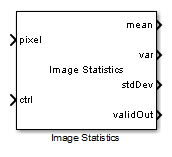# Image Statistics

Calculate mean, variance, and standard deviation

• Library:
• Vision HDL Toolbox / Statistics

•## Description

The Image Statistics block calculates the mean, variance, and standard deviation of streaming video data. Each calculation is performed over all pixels in the input region of interest (ROI). The block implements the calculations by using hardware-efficient algorithms.

This block uses a streaming pixel interface with a bus for frame control signals. This interface enables the block to operate independently of image size and format. All Vision HDL Toolbox™ blocks use the same streaming interface. The block accepts a scalar pixel value and a bus that contains five control signals. The control signals indicate the validity of each pixel and its location in the frame. To convert a frame (pixel matrix) into a pixel stream and control signals, use the Frame To Pixels block. For a full description of the interface, see Streaming Pixel Interface.

## Ports

### Input

expand all

Single image pixel in a pixel stream, specified as a scalar that represents grayscale intensity.

`double` and `single` data types are supported for simulation, but not for HDL code generation.

Data Types: `uint8` | `uint16` | `uint32` | `int8` | `int16` | `int32` | `fixed point` | `Boolean` | `double` | `single`

The `pixelcontrol` bus contains five signals. The signals describe the validity of the pixel and its location in the frame. For more information, see Pixel Control Bus.

Data Types: `bus`

### Output

expand all

The block uses full-precision arithmetic for internal calculation. At the output ports, intermediate data is cast back to the input type by using the following fixed-point settings: Rounding method = `Nearest`, and Overflow action = `Saturate`. The table shows the output word length for each calculation, relative to the input word length (IWL).

Mean VarianceStd. Deviation
IWLIWLIWL

Mean of the most recent input frame completed, returned as a scalar. The data type of mean is same as the data type of pixel.

Data Types: `uint8` | `uint16` | `fixed point`

Variance of the most recent input frame completed, returned as a scalar. The data type of var is same as the data type of pixel.

Data Types: `uint8` | `uint16` | `fixed point`

Standard deviation of the most recent input frame completed, returned as a scalar. The data type of stdDev is same as the data type of pixel.

Data Types: `uint8` | `uint16` | `fixed point`

The block sets this output to `true` when the statistic outputs for a frame are ready.

Data Types: `Boolean`

## Parameters

expand all

Select this parameter to enable the mean port and calculate the mean of each input frame.

Select this parameter to enable the var port and calculate the variance of each input frame.

Select this parameter to enable the stdDev port and calculate the standard deviation of each input frame.

## Tips

• To change the size and dimensions of the ROI, you can manipulate the input video stream control signals. See Regions of Interest.

• The number of valid pixels in the input image affects the accuracy of the mean approximation. To avoid approximation error, use an image that contains a multiple of 64n pixels, up to 64n+1 pixels, where n equals 0, 1, 2, or 3. For details of the mean approximation, see Algorithm.

• The block calculates statistics over frames up to 644 (16, 777, 216) pixels in size. This size supports HD frames.

expand all

## Extended Capabilities

(Computer Vision Toolbox) | (Computer Vision Toolbox) | (Computer Vision Toolbox) | |

### Topics

Introduced in R2015a

## SupportGet trial now